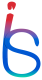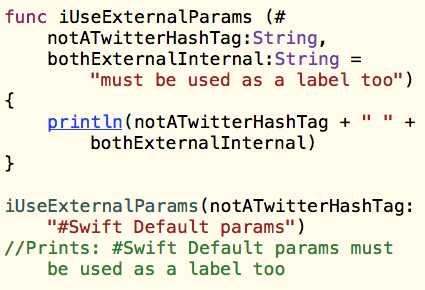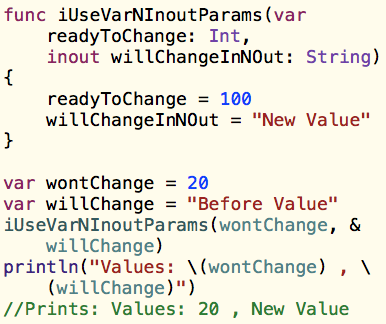# iOS Scholar's Blog

iOS Development Tutorials, Tips & Tricks and more

~~~~~~~~~~~~~~~~~~~~~~~~~~~~~~~~~~~~~~~~~~~~~~~

# Swift Functions - Shorthand External, Default, Inout and Variable Parameters

Swift Functions: Shorthand External, Default, Variable and Inout Parameters
Using hash for shorthand, modifying parameter values, reflecting them back and more...
This is second quick tutorial on Swift Functions on iOS Scholar’s Blog. The previous tutorial on Functions in Swift included Multiple Return Types and External Parameters. In this tutorial we’ll cover the Shorthand External Parameters, Variable Parameters and Inout Parameters.

Shorthand External Parameters
In the previous post, we used external parameters to improve readability and make  function usage more intuitive. However, the additional names for parameters to be used as labels can be regarded as overhead at times. This is specifically when the internal parameter name itself is apt to be used as external parameter too. In such cases, we can use the Shorthand - we simply prefix the parameter name with a hash symbol to indicate that it’s both an external and internal parameter name.

Let’s consider the example below. These two functions calculatePower and interestCalculator both have one or more parameters prefixed with hash symbols. While calling these functions, we have to use the labels for parameters which specify hash symbols.

func calculatePower(number: Double, #toThePowerOf: Int) -> Double
{
var result = 1.0
for _ in 1...toThePowerOf
{
result *= number
}
return result
}

func interestCalculator(#principal: Double, #numberOfYears: Int, #rateOfInterest: Double) ->
(simpleInterest:Double, compoundInterest:Double)
{
let simpleInterest = principal * rateOfInterest * Double(numberOfYears)
let compoundInterest = principal * (calculatePower(rateOfInterest, toThePowerOf: numberOfYears))
return (simpleInterest, compoundInterest)
}

println(interestCalculator(principal:1000, numberOfYears:1, rateOfInterest: 0.05).simpleInterest)

// prints: 50External Parameters and Default Parameters in Swift Functions

Default Parameters
In the interestCalculator function above, we could choose a default rateOfInterest by specifying a value in the function declaration itself, and then we could omit this parameter while invoking the function:

func interestCalculator(#principal: Double, rateOfInterest: Double = 0.05, #numberOfYears: Int) ->
(simpleInterest:Double, compoundInterest:Double)

Notice that we’ve not used the shorthand for external parameter - hash for rateOfInterest parameter. That’s because default parameters are implicitly external and will have to be used as labels while invoking a function - unless you choose to use the default value, in which case, the parameter is omitted altogether.

Let’s consider the following function calls. The first uses labels and specifies all parameters, while the second call doesn’t specify the second parameter, and thus uses the default value of rateOfInterest.

println(interestCalculator(principal:1000, rateOfInterest: 0.07, numberOfYears: 1).simpleInterest)
//Prints: 70.0
println(interestCalculator(principal:1000, numberOfYears: 2).compoundInterest)
//Prints: 1102.5

An important thing to note here is the order of parameters. It’s advisable to put all the default parameters towards the right. (which is intentionally not done in the example above) In other words, default parameters should be kept trailing in the list of parameters, so that, when you omit the default parameters while invoking the function, there would be no ambiguity for mapping the arguments to parameters.

Keeping the parameters with default values in the right is not just a convention, but compulsory in C/C++.

However, in Swift, even if your default parameters are not in the right, the function call will still be unambiguous, like in the example above, if just one parameter immediately following the right of default parameter have external parameters (or shorthand external parameters). In the function call above, since numberOfYears parameter is labelled, it’s clear that the rateOfInterest parameter is omitted, and since it has a default value, that will be used, making the function call unambiguous.

If for some reason, you want to escape default behavior of implicit external parameter for parameters with default values, use an underscore before the parameter name:

func interestCalculator(#principal: Double, _ rateOfInterest: Double = 0.05, #numberOfYears: Int) ->
(simpleInterest:Double, compoundInterest:Double)

The function calls will have to modified accordingly:

println
(interestCalculator(principal:1000, 0.07, numberOfYears: 1).simpleInterest)
//Prints: 70.0

Variable Parameters
The function parameters in Swift are constant (immutable) by default, which entails that you can not change the value of the parameter. Let’s consider the example below:

func doubleNumber(number: Int) -> Int
{
//    number = number * 2
// The above gives a compile time error - cannot assign to 'let' value 'number'
return (number * 2)
}

println(doubleNumber(3))
//Prints: 6
var x = 10
x = doubleNumber(x)
println(x)
//Prints: 20

The error is caused parameter number which is a “let value” by default, which means, number is a constant whose value can not be modified. But if we change the parameter’s value type to ‘var’, the function would then compile successfully:

func doubleNumber(var number: Int) -> Int
{
number = number * 2
return (number)
}

The parameter number is now a variable parameter. (The outputs remain the same, we are now returning number, instead of number * 2)Variable Parameters and Inout Parameters in Swift Functions

Inout Parameters
In the above example, we changed the parameter’s value type to variable, and thus it’s mutable within the function. However, the parameter is still a copy of the variable which is used to invoke the function. Thus, the original value remains unchanged.

In the code fragment above, we called the function doubleNumber with x as a parameter, but since the doubled value doesn’t get reflected back, we assign the doubled value to x. However, if we could reflect the modified value of x, then the returning and assigning  value are both unnecessary.

To reflect the values back in the calling function, we use “inout parameters” in Swift.

func doubleNumber(inout number: Int)
{
number *= 2
}

var x = 10
doubleNumber(&x)
println(x)
//Prints: 20
//println(doubleNumber(3))
//error: could not find an overload for 'println' that accepts the supplied arguments

Now, no value is returned from the function, we simply invoke doubleNumber() with parameter x, and the variable x gets modified accordingly. The ampersand before the parameter is compulsory - it signifies the “acceptance” of the modification that called function can make to the parameter. Looking at it the other way round, somebody reading your code, will take a look at the ampersand and know that this function takes an inout parameter.

Notice the commented error above. Since inout parameters can be modified by the called function, only variables can be sent when inout parameters are expected in the function call. Literals like “3” or “let” values can not be sent to doubleNumber()

Conclusion

In this tutorial, we discussed Shorthand External Parameters, which eliminate the requirements of separate external and internal names for parameters. Next, we checked out default parameters and some interesting stuff about how order of default parameters can make the function call ambiguous. Then, we changed the default immutable behaviour of function parameters in Swift, using default parameters. Finally, we discussed inout parameters, which let the value of parameter reflect back in the calling function / scope. That's all for this tutorial. Have fun with Swift. And please use the comments box for any feedback you would like to give.